2019/04/26 07:18

# 前言

• 第一人称模式 -- 操作就类似行人或车在行进的效果，可以通过键盘鼠标控制前进后退。
• 自动巡检模式 -- 该模式下用户不需要任何操作，场景自动前进后退来巡查当前地铁站的场景。
• 鼠标操作模式 -- 左键旋转场景，右键平移场景。

# 界面简介及效果预览

### 地铁运行效果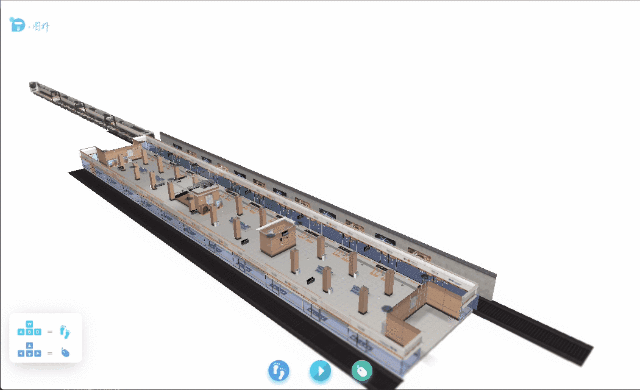### 漫游效果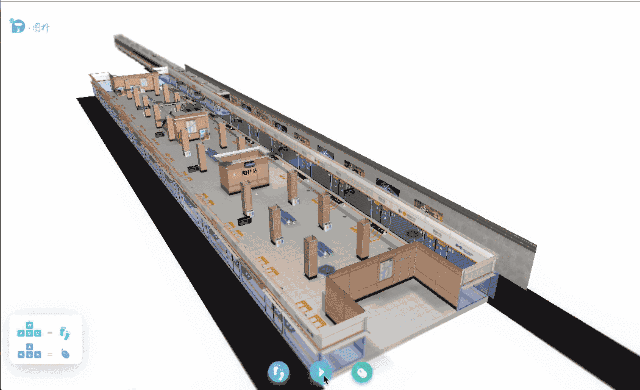### 监控设备交互效果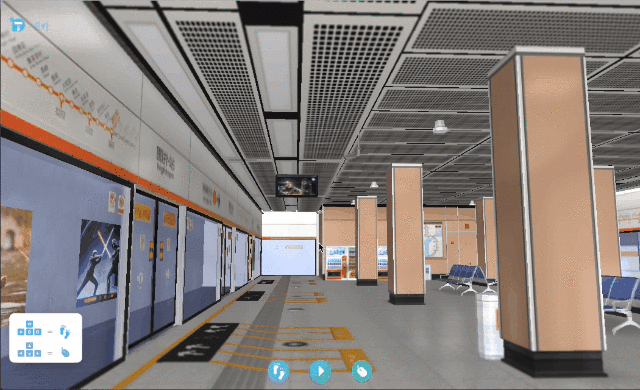# 场景搭建

// 分别为 obj 文件地址，mtl 文件地址
center: true,
// 模型是否居中，默认为 false，设置为 true 则会移动模型位置使其内容居中
r3: [0, -Math.PI / 2, 0],
// 旋转变化参数，格式为 [rx, ry, rz]
s3: [0.15, 0.15, 0.15],
// 大小变化参数，格式为 [sx, sy, sz]
finishFunc: function(modelMap, array, rawS3) {
if (modelMap) {
ht.Default.setShape3dModel('metro', array); // 注册一个名字为 metro 的模型
}
}
});


var node = new ht.Node();
node.s({
'shape3d': 'metro'
});


# 动画代码分析

### 地铁动画代码的实现分析

let metroTask = {
interval: 50,
// 每五十秒执行一次
action: (data) = >{ // 即上文所提回调函数
// 判断当时传进来的节点是否为地铁列车节点
if (data === currentMetro) {
// 获取地铁此时的 X 轴位置以及行进的方向
let currentX = data.getX(),
direction = data.a('direction');
// 根据当前的 X 轴位置获取当前的列车速度
let speed = this.getSpeedByX(currentX);
// 根据当前的 X 轴位置获取当前的列车透明度
let opacity = this.getOpacityByX(currentX);
// 判断此时 X 轴位置是否超过某个值 即地铁是在某个范围内移动
if (Math.abs(currentX) <= 5000) {
// 设置当前的透明度
opacity !== 1 ? currentMetro.s({
'shape3d.transparent': true,
'shape3d.opacity': opacity
}) : currentMetro.s({
'shape3d.transparent': false
});
// 设置当前的 X 轴位置
data.setX(currentX + direction * speed);
// 判断此时地铁的速度为 0，所以此时应该执行开门的动画
if (speed === 0) this.doorAnimation(currentMetro, direction);
}
// 右方向地铁开到头，进行复位
if (currentX > 5000 && direction === 1) {
currentMetro = leftMetro;
currentMetro.setX(5000);
}
// 左方向地铁开到头，进行复位
if (currentX < -5000 && direction === -1) {
currentMetro = rightMetro;
currentMetro.setX( - 5000);
}
}
}
};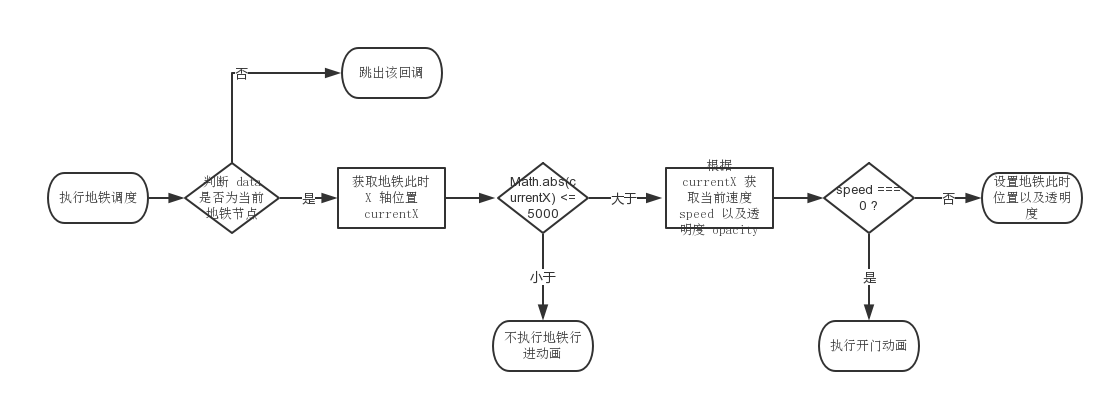currentMetro.setX(direction * 10); // 设置出站列车的位置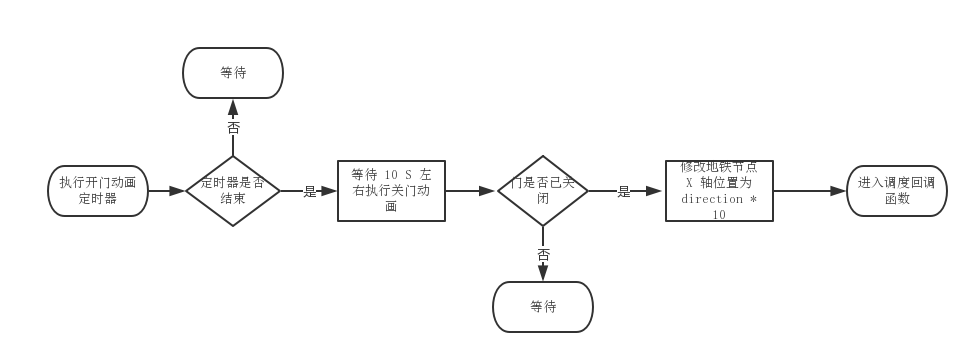### 自动巡检代码的实现分析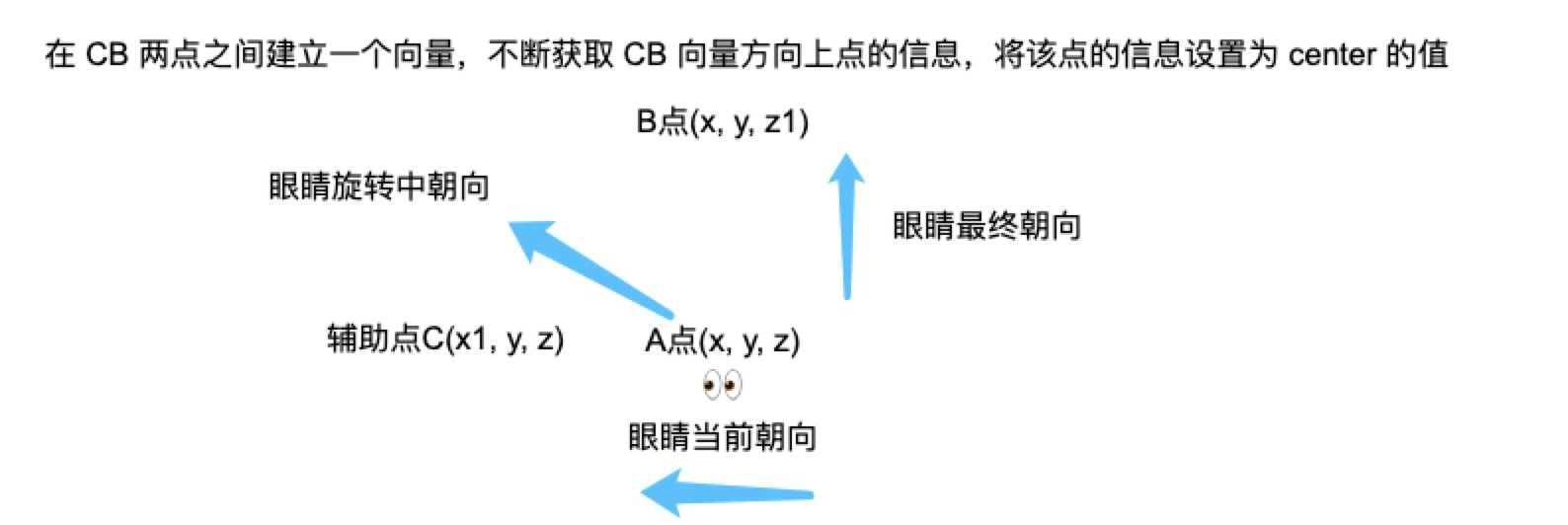rotateStep() {
// 即上图辅助点 C
let fromCenter = this.fromCenter;
// 即上图 B 点
let toCenter = this.toCenter;
// 每帧转一度
let rotateValue = this.rotateFrame || Math.PI / 180;
// 辅助点 C 与 B 点之间建立一个方向向量
let centerVector = new ht.Math.Vector2(toCenter.x - fromCenter.x, toCenter.y - fromCenter.y);
let centerVectorLength = centerVector.length();
// 此时旋转百分比
let rotatePercent = rotateValue * this.stepNum / this.curRotateVal;
if (rotatePercent >= 1) {
rotatePercent = 1;
this.stepNum = -2;
}
let newLength = rotatePercent * centerVectorLength;
centerVector.setLength(newLength);
// 获取旋转过程中 center 的点信息
let newCenterPosition = [newCenterVector.x, this.personHeight, newCenterVector.y];
// 设置当前 center 的大小
this.g3d.setCenter(newCenterPosition);
}


### 电梯动画代码的实现分析

// vs 指的是构成电梯模型所有的三角面顶点坐标数组
// 由于场景中电梯的运行方向为往对角线右上方运动，所以只需要修改 x 轴以及 y 轴坐标值
// xStep yStep 为每次电梯运动的距离
setInterval(() = >{
// i+3 是因为 vs 数组的顺序为 x, y, z 轴 所以每次 i 偏移三个单位大小
for (let i = 0, l = vs.length; i < l; i = i + 3) {
// 该顶点坐标下一个 x 轴坐标的值
let nextX = vs[i] - xStep;
// 该顶点坐标下一个 y 轴坐标的值
let nextY = vs[i + 1] + yStep;
vs[i] = nextX < -0.5 ? 0.5 - (Math.abs(nextX) - 0.5) : nextX;
vs[i + 1] = nextY > 0.5 ? -0.5 + (Math.abs(nextY) - 0.5) : nextY;
}
},
200);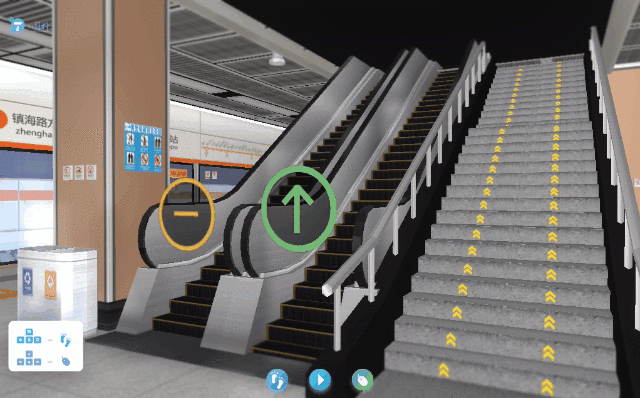# 监控功能展示及介绍

### 视频监控### 烟雾报警监控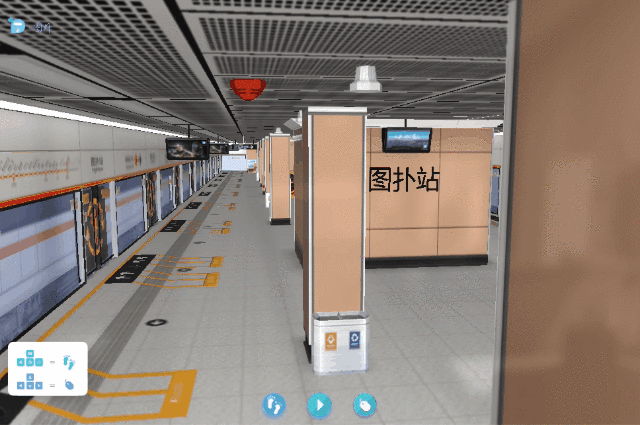### 电视列车到站时间监控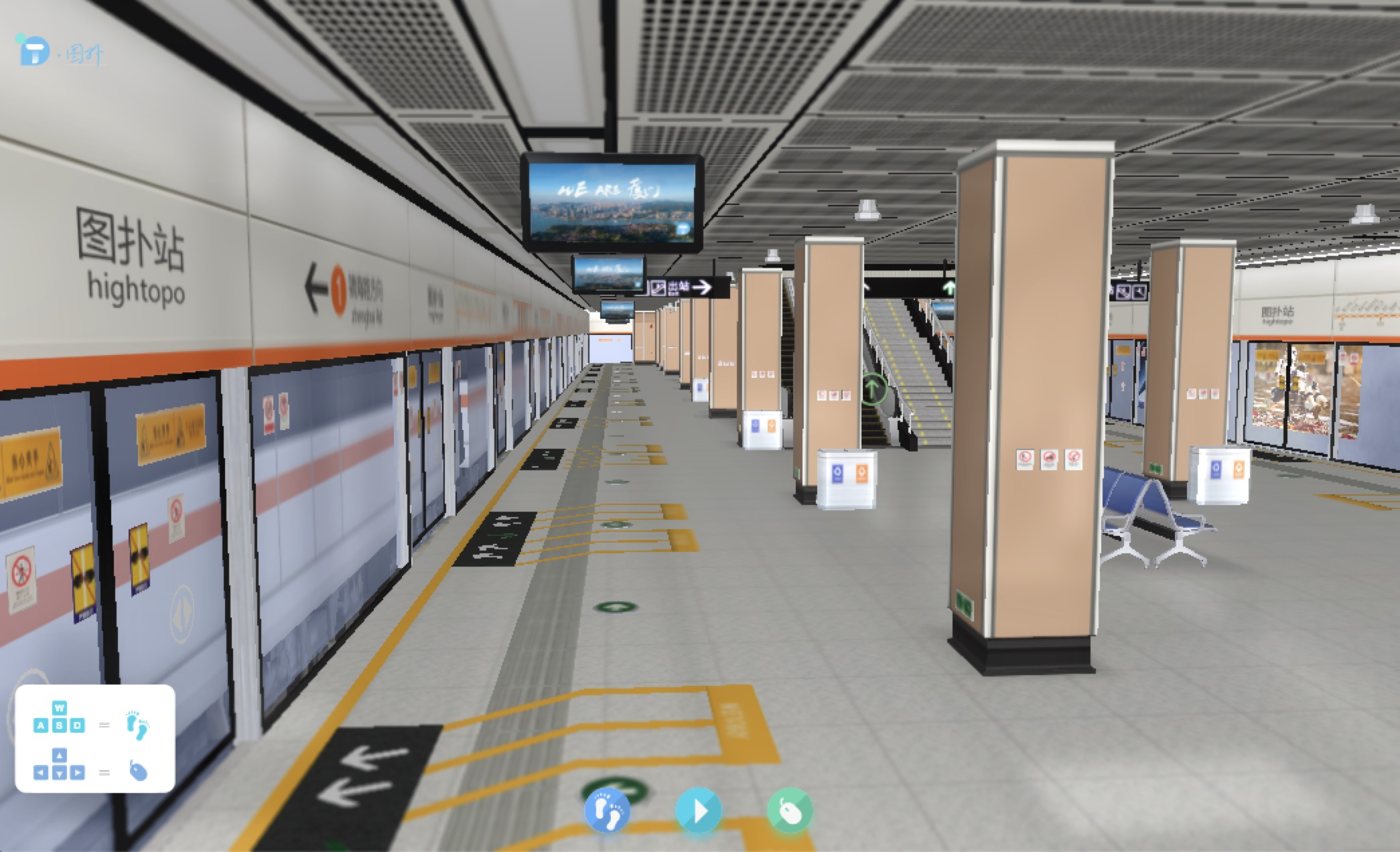### 场景监控交互

3D 场景中交互是比较简单的，主要是点击摄像头展示 2D 监控面板，在 2D 界面中主要是切换三种交互模式，三种交互模式为互斥的关系，以下是 3D 交互注册事件代码:

g3d.mi((e) = >{
let {
g2d,
dm2d
} = this;
// 为点击类型
if (e.kind === 'clickData') {
// data 为当前点击的图元
let data = e.data;
// 当前图元的 shape3d 类型
let shape3d = data.s('shape3d');
// 判断当前 shape3d 类型是否为摄像头
if (shape3d && shape3d.indexOf('摄像头') > 0) {
let cameraPanel = dm2d.getDataByTag('cameraPanel');
// toggle 切换摄像头 2d 面板
g2d.isVisible(cameraPanel) ? cameraPanel.s('2d.visible', false) : cameraPanel.s('2d.visible', true);
}
}
// 为点击 3d 场景背景类型
if (e.kind === 'clickBackground') {
let cameraPanel = dm2d.getDataByTag('cameraPanel');
// 隐藏摄像头 2d 面板
g2d.isVisible(cameraPanel) && cameraPanel.s('2d.visible', false);
}
});


# 总结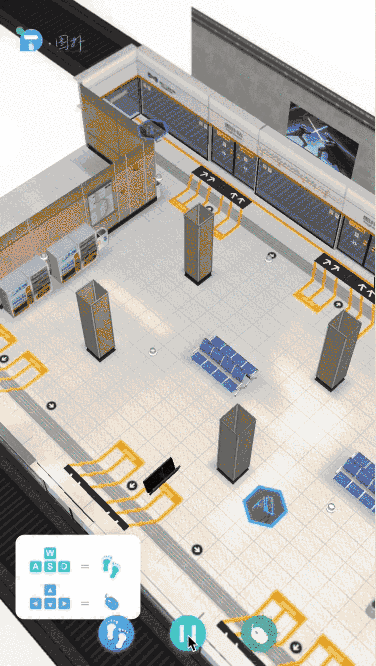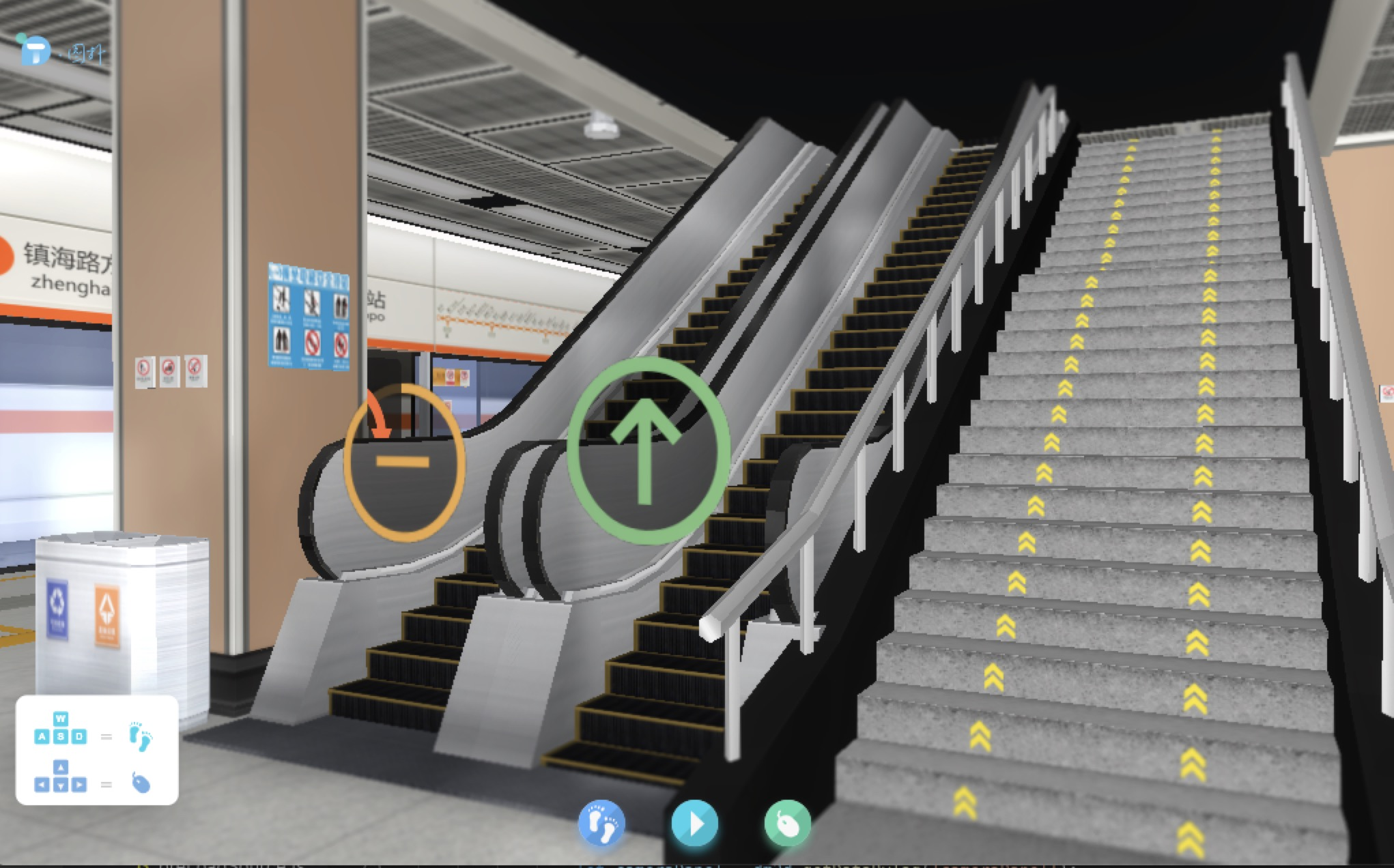### 作者的其它热门文章

0
0 收藏

0 评论
0 收藏
0